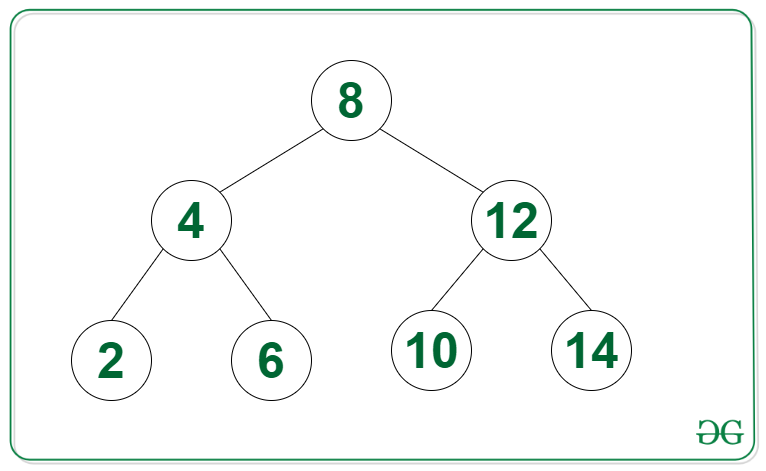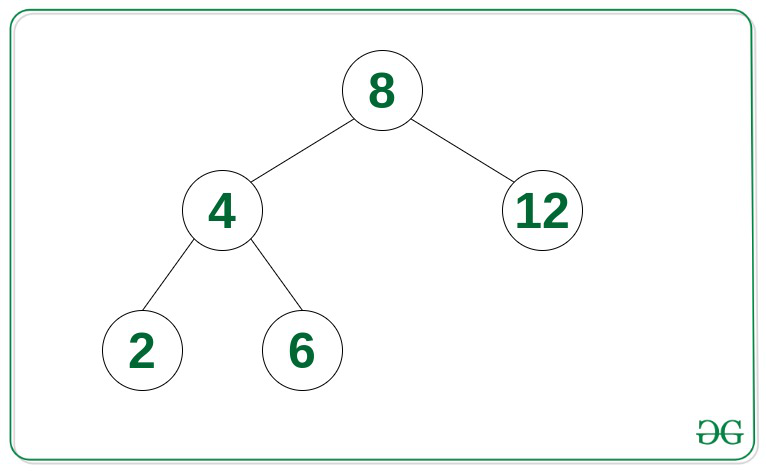# Create a wave array from the given Binary Search Tree

• Difficulty Level : Easy
• Last Updated : 25 Nov, 2021

Given a Binary Search Tree, the task is to create a wave array from the given Binary Search Tree. An array arr[0..n-1] is called a wave array if arr >= arr <= arr >= arr <= arr >= …

Examples:

Attention reader! Don’t stop learning now. Get hold of all the important DSA concepts with the DSA Self Paced Course at a student-friendly price and become industry ready.  To complete your preparation from learning a language to DS Algo and many more,  please refer Complete Interview Preparation Course.

In case you wish to attend live classes with experts, please refer DSA Live Classes for Working Professionals and Competitive Programming Live for Students.

Input:Output: 4 2 8 6 12 10 14
Explanation: The above mentioned array {4, 2, 8, 6, 12, 10, 14} is one of the many valid wave arrays.

Input:Output: 4 2 8 6 12

Approach: The given problem can be solved by the observation that the Inorder Traversal of the Binary Search Tree gives nodes in non-decreasing order. Therefore, store the inorder traversal of the given tree into a vector. Since the vector contains elements in sorted order, it can be converted into a wave array by swapping the adjacent elements for all elements in the range [0, N) using the approach discussed in this article.

Below is the implementation of the above approach:

## C++

 `// C++ program for the above approach``#include ``using` `namespace` `std;` `// Node of the Binary Search tree``struct` `Node {``    ``int` `data;``    ``Node* right;``    ``Node* left;` `    ``// Constructor``    ``Node(``int` `data)``    ``{``        ``this``->data = data;``        ``this``->left = NULL;``        ``this``->right = NULL;``    ``}``};` `// Function to convert Binary Search``// Tree into a wave Array``void` `toWaveArray(Node* root)``{``    ``// Stores the final wave array``    ``vector<``int``> waveArr;` `    ``stack s;``    ``Node* curr = root;` `    ``// Perform the Inorder traversal``    ``// of the given BST``    ``while` `(curr != NULL || s.empty() == ``false``) {` `        ``// Reach the left most Node of``        ``// the curr Node``        ``while` `(curr != NULL) {` `            ``// Place pointer to a tree node``            ``// in stack before traversing``            ``// the node's left subtree``            ``s.push(curr);``            ``curr = curr->left;``        ``}``        ``curr = s.top();``        ``s.pop();` `        ``// Insert into wave array``        ``waveArr.push_back(curr->data);` `        ``// Visit the right subtree``        ``curr = curr->right;``    ``}` `    ``// Convert sorted array into wave array``    ``for` `(``int` `i = 0;``         ``i + 1 < waveArr.size(); i += 2) {``        ``swap(waveArr[i], waveArr[i + 1]);``    ``}` `    ``// Print the answer``    ``for` `(``int` `i = 0; i < waveArr.size(); i++) {``        ``cout << waveArr[i] << ``" "``;``    ``}``}` `// Driver Code``int` `main()``{``    ``Node* root = ``new` `Node(8);``    ``root->left = ``new` `Node(4);``    ``root->right = ``new` `Node(12);``    ``root->right->left = ``new` `Node(10);``    ``root->right->right = ``new` `Node(14);``    ``root->left->left = ``new` `Node(2);``    ``root->left->right = ``new` `Node(6);` `    ``toWaveArray(root);` `    ``return` `0;``}`

## Java

 `// Java program for the above approach``import` `java.util.*;` `class` `GFG{` `// Node of the Binary Search tree``static` `class` `Node {``    ``int` `data;``    ``Node right;``    ``Node left;` `    ``// Constructor``    ``Node(``int` `data)``    ``{``        ``this``.data = data;``        ``this``.left = ``null``;``        ``this``.right = ``null``;``    ``}``};` `// Function to convert Binary Search``// Tree into a wave Array``static` `void` `toWaveArray(Node root)``{``  ` `    ``// Stores the final wave array``    ``Vector waveArr = ``new` `Vector<>();` `    ``Stack s = ``new` `Stack<>();``    ``Node curr = root;` `    ``// Perform the Inorder traversal``    ``// of the given BST``    ``while` `(curr != ``null` `|| s.isEmpty() == ``false``) {` `        ``// Reach the left most Node of``        ``// the curr Node``        ``while` `(curr != ``null``) {` `            ``// Place pointer to a tree node``            ``// in stack before traversing``            ``// the node's left subtree``            ``s.add(curr);``            ``curr = curr.left;``        ``}``        ``curr = s.peek();``        ``s.pop();` `        ``// Insert into wave array``        ``waveArr.add(curr.data);` `        ``// Visit the right subtree``        ``curr = curr.right;``    ``}` `    ``// Convert sorted array into wave array``    ``for` `(``int` `i = ``0``;   i + ``1` `< waveArr.size(); i += ``2``) {``        ``int` `t = waveArr.get(i);``        ``waveArr.set(i, waveArr.get(i+``1``));``        ``waveArr.set(i+``1``, t);``        ` `    ``}` `    ``// Print the answer``    ``for` `(``int` `i = ``0``; i < waveArr.size(); i++) {``        ``System.out.print(waveArr.get(i)+ ``" "``);``    ``}``}` `// Driver Code``public` `static` `void` `main(String[] args)``{``    ``Node root = ``new` `Node(``8``);``    ``root.left = ``new` `Node(``4``);``    ``root.right = ``new` `Node(``12``);``    ``root.right.left = ``new` `Node(``10``);``    ``root.right.right = ``new` `Node(``14``);``    ``root.left.left = ``new` `Node(``2``);``    ``root.left.right = ``new` `Node(``6``);` `    ``toWaveArray(root);` `}``}` `// This code is contributed by umadevi9616`

## Javascript

 ``
Output:
`4 2 8 6 12 10 14`

Time Complexity: O(N)
Auxiliary Space: O(N)

My Personal Notes arrow_drop_up